# NCERT solutions for Mathematics Exemplar Class 12 chapter 12 - Linear Programming [Latest edition]

#### Chapters## Chapter 12: Linear Programming

Solved ExamplesExercise
Solved Examples [Pages 243 - 249]

### NCERT solutions for Mathematics Exemplar Class 12 Chapter 12 Linear Programming Solved Examples [Pages 243 - 249]

Solved Examples | Q 1 | Page 243

Determine the maximum value of Z = 4x + 3y if the feasible region for an LPP is shown in figure

Solved Examples | Q 2 | Page 243

Determine the minimum value of Z = 3x + 2y (if any), if the feasible region for an LPP is shown in Figue.

Solved Examples | Q 3 | Page 244

Solve the following LPP graphically:
Maximise Z = 2x + 3y, subject to x + y ≤ 4, x ≥ 0, y ≥ 0

Solved Examples | Q 4 | Page 245

A manufacturing company makes two types of television sets; one is black and white and the other is colour. The company has resources to make at most 300 sets a week. It takes Rs 1800 to make a black and white set and Rs 2700 to make a coloured set. The company can spend not more than Rs 648000 a week to make television sets. If it makes a profit of Rs 510 per black and white set and Rs 675 per coloured set, how many sets of each type should be produced so that the company has maximum profit? Formulate this problem as a LPP given that the objective is to maximise the profit

Solved Examples | Q 5 | Page 246

A manufacturing company makes two types of television sets; one is black and white and the other is colour. The company has resources to make at most 300 sets a week. It takes Rs 1800 to make a black and white set and Rs 2700 to make a coloured set. The company can spend not more than Rs 648000 a week to make television sets. If it makes a profit of Rs 510 per black and white set and Rs 675 per coloured set, how many sets of each type should be produced so that the company has maximum profit? Formulate this problem as a LPP given that the objective is to maximise the profit.

Solved Examples | Q 6 | Page 247

Minimise Z = 3x + 5y subject to the constraints:
x + 2y ≥ 10
x + y ≥ 6
3x + y ≥ 8
x, y ≥ 0

#### Objective Type Questions

Solved Examples | Q 7 | Page 248

The corner points of the feasible region determined by the system of linear constraints are (0, 10), (5, 5), (15, 15), (0, 20). Let Z = px + qy, where p, q > 0. Condition on p and q so that the maximum of Z occurs at both the points (15, 15) and (0, 20) is ______.

• p = q

• p = 2q

• q = 2p

• q = 3p

Solved Examples | Q 8 | Page 248

Feasible region (shaded) for a LPP is shown in the Figure Minimum of Z = 4x + 3y occurs at the point ______.

• (0, 8)

• (2, 5)

• (4, 3)

• (9, 0)

#### Fill in the blanks 9 to 10

Solved Examples | Q 9 | Page 249

In a LPP, the linear function which has to be maximised or minimised is called a linear ______ function.

Solved Examples | Q 10 | Page 249

The common region determined by all the linear constraints of a LPP is called the ______ region.

Solved Examples | Q 11 | Page 249

If the feasible region for a linear programming problem is bounded, then the objective function Z = ax + by has both a maximum and a minimum value on R.

• True

• False

Solved Examples | Q 12 | Page 249

The minimum value of the objective function Z = ax + by in a linear programming problem always occurs at only one corner point of the feasible region

• True

• False

Exercise [Pages 250 - 257]

### NCERT solutions for Mathematics Exemplar Class 12 Chapter 12 Linear Programming Exercise [Pages 250 - 257]

Exercise | Q 1 | Page 250

Determine the maximum value of Z = 11x + 7y subject to the constraints : 2x + y ≤ 6, x ≤ 2, x ≥ 0, y ≥ 0.

Exercise | Q 2 | Page 250

Maximise Z = 3x + 4y, subject to the constraints: x + y ≤ 1, x ≥ 0, y ≥ 0

Exercise | Q 3 | Page 250

Maximise the function Z = 11x + 7y, subject to the constraints: x ≤ 3, y ≤ 2, x ≥ 0, y ≥ 0.

Exercise | Q 4 | Page 250

Minimise Z = 13x – 15y subject to the constraints: x + y ≤ 7, 2x – 3y + 6 ≥ 0, x ≥ 0, y ≥ 0

Exercise | Q 5 | Page 250

Determine the maximum value of Z = 3x + 4y if the feasible region (shaded) for a LPP is shown in Figure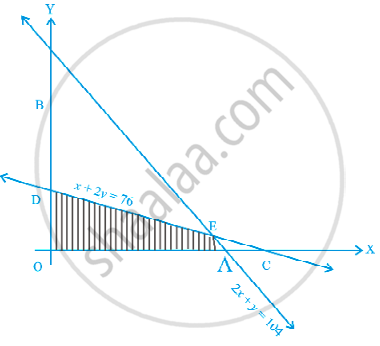Exercise | Q 6 | Page 250

Feasible region (shaded) for a LPP is shown in Figure. Maximise Z = 5x + 7y.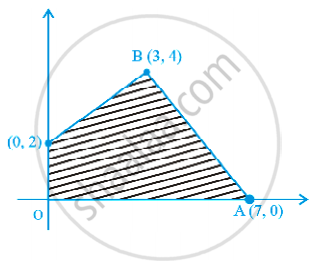Exercise | Q 7 | Page 251

The feasible region for a LPP is shown in Figure. Find the minimum value of Z = 11x + 7y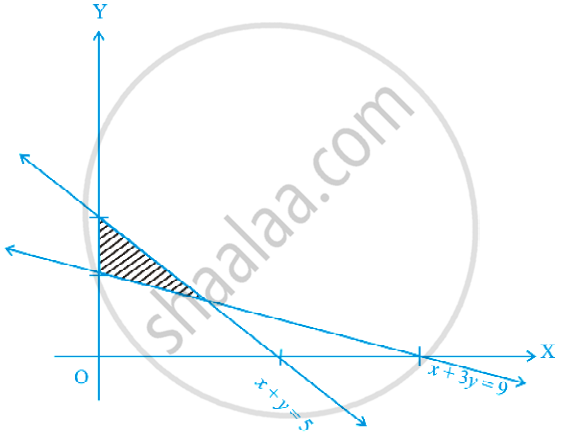Exercise | Q 8 | Page 251

Refer to Exercise 7 above. Find the maximum value of Z.

Exercise | Q 9 | Page 251

The feasible region for a LPP is shown in figure. Evaluate Z = 4x + y at each of the corner points of this region. Find the minimum value of Z, if it exists.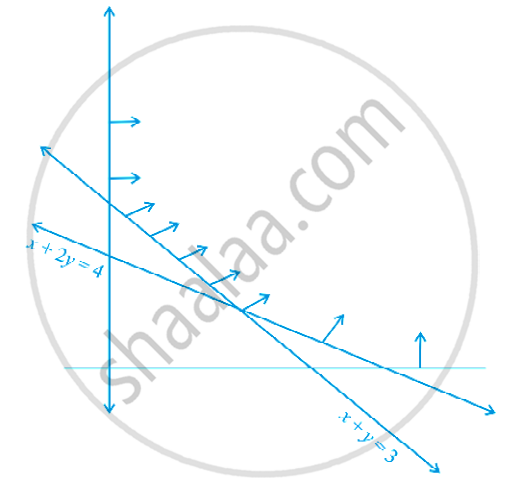Exercise | Q 10 | Page 252

In figure, the feasible region (shaded) for a LPP is shown. Determine the maximum and minimum value of Z = x + 2y.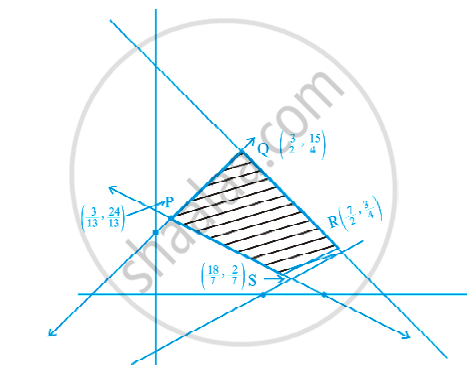Exercise | Q 11 | Page 252

A manufacturer of electronic circuits has a stock of 200 resistors, 120 transistors and 150 capacitors and is required to produce two types of circuits A and B. Type A requires 20 resistors, 10 transistors and 10 capacitors. Type B requires 10 resistors, 20 transistors and 30 capacitors. If the profit on type A circuit is Rs 50 and that on type B circuit is Rs 60, formulate this problem as a LPP so that the manufacturer can maximise his profit.

Exercise | Q 12 | Page 252

A firm has to transport 1200 packages using large vans which can carry 200 packages each and small vans which can take 80 packages each. The cost for engaging each large van is Rs 400 and each small van is Rs 200. Not more than Rs 3000 is to be spent on the job and the number of large vans can not exceed the number of small vans. Formulate this problem as a LPP given that the objective is to minimise cost.

Exercise | Q 13 | Page 252

A company manufactures two types of screws A and B. All the screws have to pass through a threading machine and a slotting machine. A box of Type A screws requires 2 minutes on the threading machine and 3 minutes on the slotting machine. A box of type B screws requires 8 minutes of threading on the threading machine and 2 minutes on the slotting machine. In a week, each machine is available for 60 hours. On selling these screws, the company gets a profit of Rs 100 per box on type A screws and Rs 170 per box on type B screws. Formulate this problem as a LPP given that the objective is to maximise profit.

Exercise | Q 14 | Page 253

A company manufactures two types of sweaters : type A and type B. It costs Rs 360 to make a type A sweater and Rs 120 to make a type B sweater. The company can make at most 300 sweaters and spend at most Rs 72000 a day. The number of sweaters of type B cannot exceed the number of sweaters of type A by more than 100. The company makes a profit of Rs 200 for each sweater of type A and Rs 120 for every sweater of type B.
Formulate this problem as a LPP to maximise the profit to the company.

Exercise | Q 15 | Page 253

A man rides his motorcycle at the speed of 50 km/hour. He has to spend Rs 2 per km on petrol. If he rides it at a faster speed of 80 km/hour, the petrol cost increases to Rs 3 per km. He has atmost Rs 120 to spend on petrol and one hour’s time. He wishes to find the maximum distance that he can travel. Express this problem as a linear programming problem

Exercise | Q 16 | Page 253

Refer to question 11. How many of circuits of Type A and of Type B, should be produced by the manufacturer so as to maximise his profit? Determine the maximum profit.

Exercise | Q 17 | Page 253

Refer to quastion 12. What will be the minimum cost?

Exercise | Q 18 | Page 253

Refer to question 13. Solve the linear programming problem and determine the maximum profit to the manufacturer

Exercise | Q 19 | Page 253

Refer to question 14. How many sweaters of each type should the company make in a day to get a maximum profit? What is the maximum profit.

Exercise | Q 20 | Page 253

Refer to question 15. Determine the maximum distance that the man can travel.

Exercise | Q 20 | Page 253

Refer to question 15. Determine the maximum distance that the man can travel.

Exercise | Q 21 | Page 253

Maximise Z = x + y subject to x + 4y ≤ 8, 2x + 3y ≤ 12, 3x + y ≤ 9, x ≥ 0, y ≥ 0.

Exercise | Q 22 | Page 253

A manufacturer produces two Models of bikes-Model X and Model Y. Model X takes a 6 man-hours to make per unit, while Model Y takes 10 man-hours per unit. There is a total of 450 man-hour available per week. Handling and Marketing costs are Rs 2000 and Rs 1000 per unit for Models X and Y respectively. The total funds available for these purposes are Rs 80,000 per week. Profits per unit for Models X and Y are Rs 1000 and Rs 500, respectively. How many bikes of each model should the manufacturer produce so as to yield a maximum profit? Find the maximum profit.

Exercise | Q 23 | Page 254

In order to supplement daily diet, a person wishes to take some X and some wishes Y tablets. The contents of iron, calcium and vitamins in X and Y (in milligrams per tablet) are given as below:

 Tablets Iron Calcium Vitamin X 6 3 2 Y 2 3 4

The person needs atleast 18 milligrams of iron, 21 milligrams of calcium and 16 milligrams of vitamin. The price of each tablet of X and Y is Rs 2 and Rs 1 respectively. How many tablets of each should the person take in order to satisfy the above requirement at the minimum cost?

Exercise | Q 24 | Page 254

A company makes 3 model of calculators: A, B and C at factory I and factory II. The company has orders for at least 6400 calculators of model A, 4000 calculator of model B and 4800 calculator of model C. At factory I, 50 calculators of model A, 50 of model B and 30 of model C are made every day; at factory II, 40 calculators of model A, 20 of model B and 40 of model C are made everyday. It costs Rs 12000 and Rs 15000 each day to operate factory I and II, respectively. Find the number of days each factory should operate to minimise the operating costs and still meet the demand.

Exercise | Q 25 | Page 254

Maximise and Minimise Z = 3x – 4y subject to x – 2y ≤ 0, – 3x + y ≤ 4, x – y ≤ 6, x, y ≥ 0

#### Objective Type Questions from 26 to 34

Exercise | Q 26 | Page 254

The corner points of the feasible region determined by the system of linear constraints are (0, 0), (0, 40), (20, 40), (60, 20), (60, 0). The objective function is Z = 4x + 3y ______.

Compare the quantity in Column A and Column B

 Column A Column B Maximum of Z 325
• The quantity in column A is greater

• The quantity in column B is greater

• The two quantities are equal

• The relationship can not be determined on the basis of the information supplied

Exercise | Q 27 | Page 255

The feasible solution for a LPP is shown in Figure. Let Z = 3x – 4y be the objective function. Minimum of Z occurs at ______.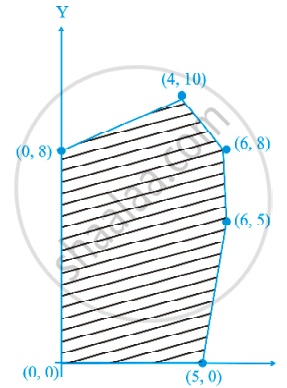• (0, 0)

• (0, 8)

• (5, 0)

• (4, 10)

Exercise | Q 28 | Page 255

Refer to Question 27. Maximum of Z occurs at ______.

• (5, 0)

• (6, 5)

• (6, 8)

• (4, 10)

Exercise | Q 29 | Page 255

Refer to Question 27. (Maximum value of Z + Minimum value of Z) is equal to ______.

• 13

• 1

• – 13

• – 17

Exercise | Q 30 | Page 255

The feasible region for an LPP is shown in the figure. Let F = 3x – 4y be the objective function. Maximum value of F is ______.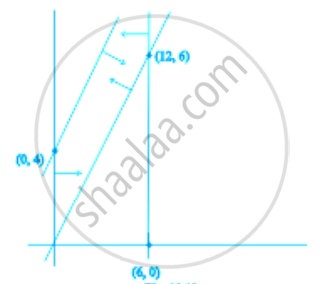• 0

• 8

• 12

• – 18

Exercise | Q 31 | Page 256

Refer to Question 30. Minimum value of F is ______.

• 0

• – 16

• 12

• Does not exist

Exercise | Q 32 | Page 256

Corner points of the feasible region for an LPP are (0, 2), (3, 0), (6, 0), (6, 8) and (0, 5). Let F = 4x + 6y be the objective function. The Minimum value of F occurs at  ______.

• (0, 2) only

• (3, 0) only

• The midpoint of the line segment joining the points (0, 2) and (3, 0) only

• Any point on the line segment joining the points (0, 2) and (3, 0).

Exercise | Q 33 | Page 256

Refer to Question 32, Maximum of F – Minimum of F = ______.

• 60

• 48

• 42

• 18

Exercise | Q 34 | Page 256

Corner points of the feasible region determined by the system of linear constraints are (0, 3), (1, 1) and (3, 0). Let Z = px+qy, where p, q > 0. Condition on p and q so that the minimum of Z occurs at (3, 0) and (1, 1) is ______.

• p = 2q

• p = "q"/2

• p = 3q

• p = q

#### Fill in the blanks 35 to 41

Exercise | Q 35 | Page 257

In a LPP, the linear inequalities or restrictions on the variables are called ____________.

Exercise | Q 36 | Page 257

In a LPP, the objective function is always ______.

Exercise | Q 37 | Page 257

If the feasible region for a LPP is ______ then the optimal value of the objective function Z = ax + by may or may not exist.

Exercise | Q 38 | Page 257

In a LPP if the objective function Z = ax + by has the same maximum value on two corner points of the feasible region, then every point on the line segment joining these two points give the same ______ value.

Exercise | Q 39 | Page 257

A feasible region of a system of linear inequalities is said to be ______ if it can be enclosed within a circle.

Exercise | Q 40 | Page 257

A corner point of a feasible region is a point in the region which is the ______ of two boundary lines.

Exercise | Q 41 | Page 257

The feasible region for an LPP is always a ______ polygon.

#### State whether the following statement is True or False: 42 to 45

Exercise | Q 42 | Page 257

If the feasible region for a LPP is unbounded, maximum or minimum of the objective function Z = ax + by may or may not exist.

• True

• False

Exercise | Q 43 | Page 257

Maximum value of the objective function Z = ax + by in a LPP always occurs at only one corner point of the feasible region.

• True

• False

Exercise | Q 44 | Page 257

In a LPP, the minimum value of the objective function Z = ax + by is always 0 if the origin is one of the corner point of the feasible region.

• False

• True

Exercise | Q 45 | Page 257

In a LPP, the maximum value of the objective function Z = ax + by is always finite.

• True

• False

## Chapter 12: Linear Programming

Solved ExamplesExercise## NCERT solutions for Mathematics Exemplar Class 12 chapter 12 - Linear Programming

NCERT solutions for Mathematics Exemplar Class 12 chapter 12 (Linear Programming) include all questions with solution and detail explanation. This will clear students doubts about any question and improve application skills while preparing for board exams. The detailed, step-by-step solutions will help you understand the concepts better and clear your confusions, if any. Shaalaa.com has the CBSE Mathematics Exemplar Class 12 solutions in a manner that help students grasp basic concepts better and faster.

Further, we at Shaalaa.com provide such solutions so that students can prepare for written exams. NCERT textbook solutions can be a core help for self-study and acts as a perfect self-help guidance for students.

Concepts covered in Mathematics Exemplar Class 12 chapter 12 Linear Programming are Introduction of Linear Programming, Mathematical Formulation of Linear Programming Problem, Different Types of Linear Programming Problems, Graphical Method of Solving Linear Programming Problems, Linear Programming Problem and Its Mathematical Formulation.

Using NCERT Class 12 solutions Linear Programming exercise by students are an easy way to prepare for the exams, as they involve solutions arranged chapter-wise also page wise. The questions involved in NCERT Solutions are important questions that can be asked in the final exam. Maximum students of CBSE Class 12 prefer NCERT Textbook Solutions to score more in exam.

Get the free view of chapter 12 Linear Programming Class 12 extra questions for Mathematics Exemplar Class 12 and can use Shaalaa.com to keep it handy for your exam preparation